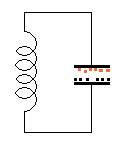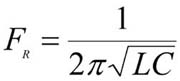# Method of Pulsing an Asymmetrical Capacitor Thruster

Comment relevant to the discussion of the Lafforgue thruster in Secrets of Antigravity Propulsion pp. 373-374

Most likely the Lafforgue thruster loses its thrust once it becomes fully charged due to the opposing thrust vector developed by its polarized dielectric. Hence, like Brown’s electrokinetic apparatus, it may need to be repeatedly charged and discharged to create a continuing thrust effect. One way to do this would be to use pulsed DC. However, AC can also be used. Both of these asymmetrical capacitors would maintain a unidirectional thrust toward the larger electrode even when energized with AC. Unlike the electrogravitic thrust which is directed toward the positive electrode, the unbalanced forces developed in an asymmetrical capacitor are charge independent.So one may take advantage of this and connect the asymmetrical capacitor in parallel with a coil to form a tank circuit. This allows the capacitor-coil circuit to resonate and alternate the charge polarity on the plates at the tank’s natural resonant frequency. This would help conserve the energy of the system. The rate of energy loss from such a tank circuit would depend on the circuit’s Q-factor. If for example, the circuit had Q = 100, it would lose 1 percent of its energy with each cycle of oscillation. So compared to the situation of repeatedly charging and discharging the asymmetrical capacitor with DC, when operated in an AC mode as a tank circuit its power consumption would be reduced by a factor of 100. In AC charging mode there would no longer be the added contribution of an electrogravitic thrust, but the advantages of saving power would greatly outweigh this disadvantage.

Let us consider the case of 30 Lafforgue thrusters delivering 20 metric tons of force. If these thrusters were to have a combined capacitance of about 30 microfarads and were to be charged to 100 kilovolts DC once every second, they would draw 300 kilowatts of power. This would project a thrust-to-power ratio of about 670 newtons per kilowatt, or 45 times that of a jet engine (as was estimated in the third edition of Secrets of Antigravity Propulsion). On the other hand, if configured as a tank circuit and induced to oscillate resonantly, its power consumption would drop to 3 kilowatts and its thrust-to-power ratio would now rise to 67,000 newtons per kilowatt, or 4500 times that of a jet engine.

When calculating the coefficient of performance of a Lafforgue motor: Considering the example discussed on p. 374, if the motor’s Lafforgue capacitors were being charged and discharged once per second, rather than being powered with a steady DC potential, the motor would require 40 kilowatts of power for its operation. Hence its output power would exceed its input power by a factor of 125. However, if operated in AC mode as a tank circuit with Q = 100, its power consumption would drop to 400 watts resulting in an overunity coefficient of performance of 12,500.